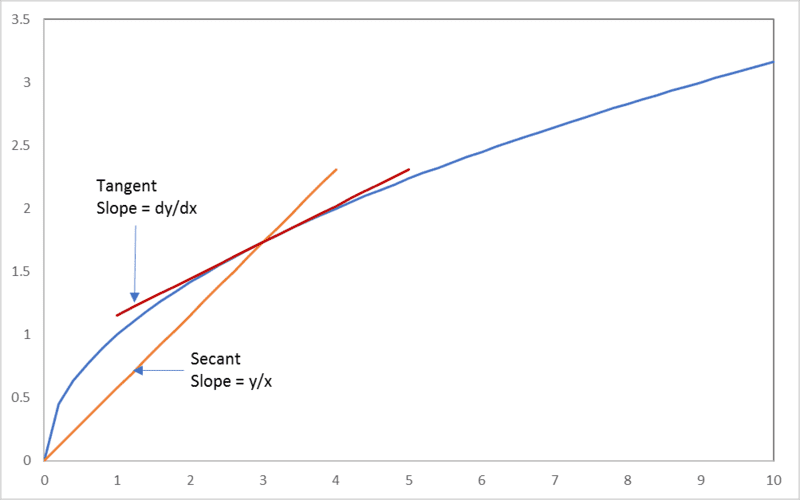# Integration and derivation

• SHOORY

## Homework Statement

is dx/dy= x/y
if yes can i use it always

## Homework Equations

for example in a circle
dA/dtheta=A/360

## The Attempt at a Solution

if its right sometimes what are the conditions of using it

Generally, no it is not. (You can integrate the expression to find the conditions under which it is true.)

•Delta2 and SHOORY
Generally, no it is not.
And it is quite easy to find counter-examples. For example, let ##y = x^2##. Then ##dy/dx = 2x \neq y/x = x##.

•SHOORY
And it is quite easy to find counter-examples. For example, let ##y = x^2##. Then ##dy/dx = 2x \neq y/x = x##.
ok thank you

See the graph below for an illustration. The secant is the line through the origin that cuts the curve at (x,y); its slope is y/x. The tangent is the line that touches the curve at (x,y); its slope is dy/dx, the instantaneous slope of the curve at (x,y), or the rate of change of y with x at that point.#### Attachments

•SHOORY, Orodruin and Delta2